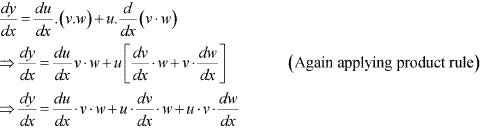# Class 12 Maths NCERT Solutions for Chapter 5 Continuity and Differentiability Exercise 5.5### Continuity and Differentiability Exercise 5.5 Solutions

1. Differentiate the function with respect to x.
cos x . cos 2x . cos 3x

Solution

Let y = cos x . cos 2x .cos 3x
Taking logarithm on both the sides, we obtain
log y = log(cos x . cos 2x . cos 3x)
⇒ log y = log(cos x) + log(cos 2x) + log(cos 3x)
Differentiating both sides with respect to x, we obtain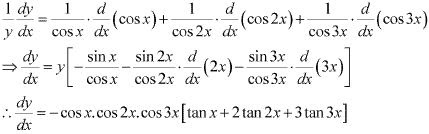2. Differentiate the function with respect to x.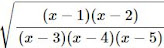Solution

Let y =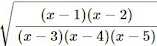Taking logarithm on both the sides, we obtain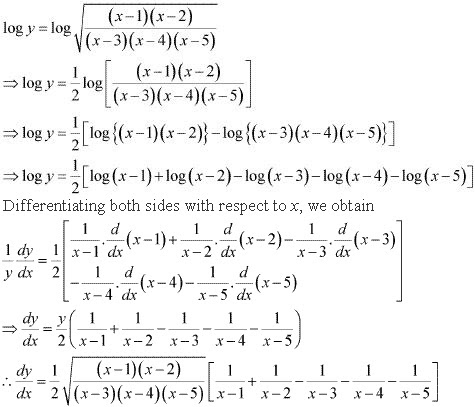3. Differentiate the function with respect to x.
(log x)cos x

Solution

Let y = (log x)cos x
Taking logarithm on both the sides, we obtain
log y = cos x. log (log x)
Differentiating both sides with respect to x, we obtain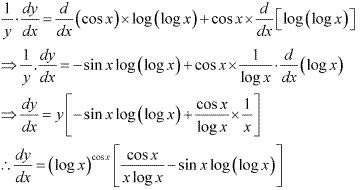4. Differentiate the function with respect to x.
x- 2sin x

Solution

Let y = x- 2sin x

Also, let xx = u and 2sin x = v
∴ y = u - v
⇒ dy/dx = (du/dx ) - dv/dx
u = xx
Taking logarithm on both the sides, we obtain
log u = x log x
Differentiating both sides with respect to x, we obtain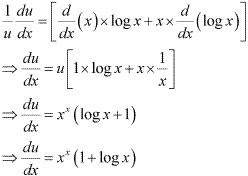v = 2sin x
Taking logarithm on both the sides with respect to x, we obtain
log v = sin x. log 2
Differentiating both sides with respect to x, we obtain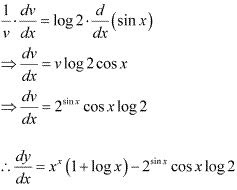5. Differentiate the function with respect to x.
(x +3)2 . (x + 4)3 . (x + 5)4

Solution

Let y = (x +3)2 . (x + 4)3 . (x + 5)4
Taking logarithm on both the sides, we obtain
log y = log(x +3)2 + log(x + 4)3 + log(x + 5)4
⇒ log y = 2log(x + 3) + 3 log(x + 4) + 4 log(x + 5)
Differentiating both sides with respect to x, we obtain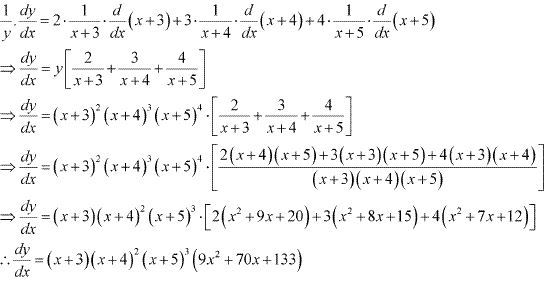6. Differentiate the function with respect to x.
(x + 1/x)x + x(1 + 1/x)
Solution
Let y = (x + 1/x)x + x(1 + 1/x)
Also, let u = (x + 1/x)x and v = x(1 + 1/x)
∴ y = u + v
⇒ dy/dx = du/dx + dv/dx  ...(1)
Then u = (x + 1/x)x
⇒ log u = log (x + 1/x)x
⇒ log u = x log (x + 1/x)
Differentiating both sides with respect to x, we obtain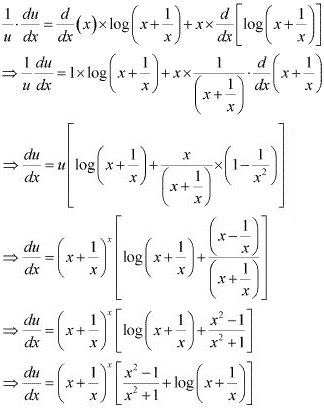v = x(1 + 1/x)
⇒ log v = log [x(1 + 1/x) )
⇒ log v = (1 + 1/x) log x
Differentiating both sides with respect to x, we obtain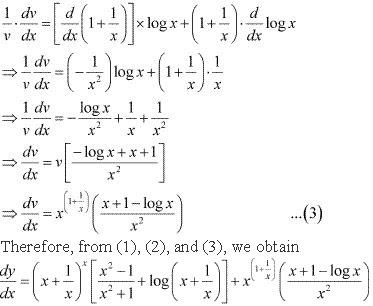7. Differentiate the function with respect to x.
(log x)x + xlog x
Solution
Let y = (log x)x + xlog x
Also, let u = (log x)x and v = xlog x
∴ y = u + v
⇒ dy/dx = du/dx + dv/dx  ...(1)
u = (log x)x
⇒ log u = log [(log x)x ]
⇒ log u =  x log (log x)
Differentiating both sides with respect to x, we obtain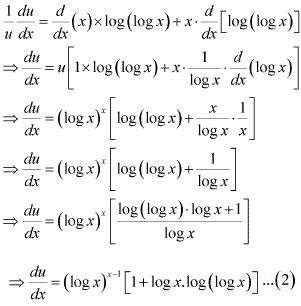v = xlog x
⇒ log v = log (xlog x )
⇒ log v = logx . logx = (log x)2
Differentiating both sides with respect to x, we obtain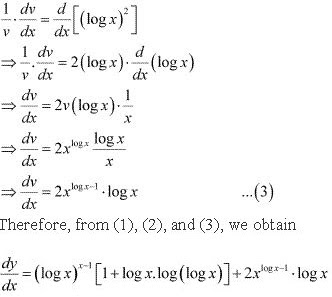8. Differentiate the function with respect to x.
(sin x)x + sin-1 √x
Solution
Let y = (sin x)x + sin-1 √x
Also, let u = (sin x)x and v = sin-1 √x
∴ y = u + v
⇒ dy/dx = du/dx + dv/dx ...(1)
u = (sin x)x
⇒ log u = log (sin x)x
⇒ log u = x log(sin x)
Differentiating both sides with respect to x, we obtain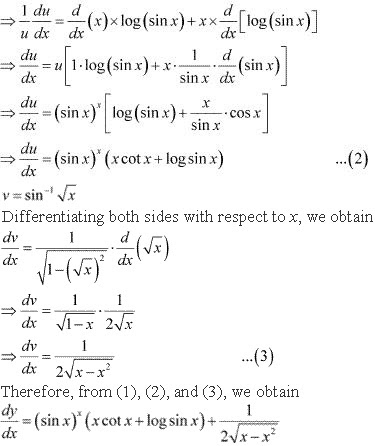9. Differentiate the function with respect to x.
xsin x + (sin x)cos x
Solution
Let y = xsin x + (sin x)cos x
Also, let u = xsin x and v = (sin x)cos x
∴ y = u + v
⇒ dy/dx = du/dx + dv/dx  ...(1)
u = xsin x
⇒ log u = log(xsin x )
⇒ log u = sin x logx
Differentiating both sides with respect to x, we obtain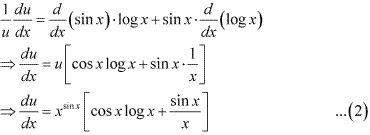v = (sin x)cos x
⇒ log v = log(sin x)cos x
⇒ log v = cos x log (sin x)
Differentiating both sides with respect to x, we obtain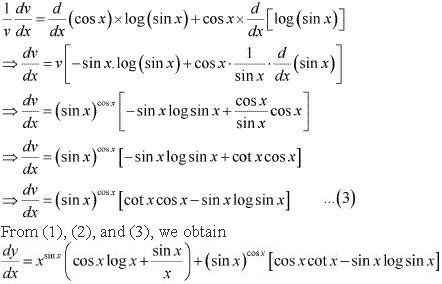10. Differentiate the function with respect to x.
xx cos x  + (x2 + 1)/(x2 - 1)
Solution
Let y = xx cos x  + (x2 + 1)/(x2 - 1)
Also, let u = xx cos x  and v = (x2 + 1)/(x2 - 1)
∴ y = u + v
⇒  dy/dx = du/dx + dv/dx  ...(1)
u = xx cos x
⇒ log u = log (xx cos x  )
⇒ log u = x cos x log x
Differentiating both sides with respect to x, we obtain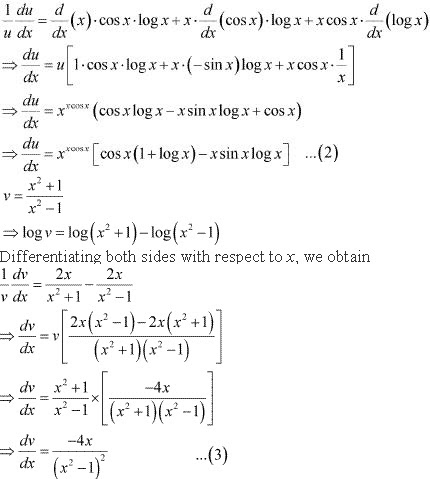From (1), (2), and (3), we obtain
dy/dx = xx cos x  [cos x(1 + log x) - x sin x log x] - 4x/(x2 - 1)2

11. Differentiate the function with respect to x.
(x cos x)x +  (x sin x)1/x
Solution
Let y =  (x cos x)x +  (x sin x)1/x
Also, let u = (x cos x)x and v =  (x sin x)1/x
∴ y = u + v
⇒ dy/dx = du/dx + dv/dx  ...(1)
u = (x cos x)x
⇒ log u = log (x cos x)x
⇒ log u = x log(x cos x)
⇒ log u = x[log x + log cos x]
⇒ log u = x log x + x log cos x
Differentiating both sides with respect to x, we obtain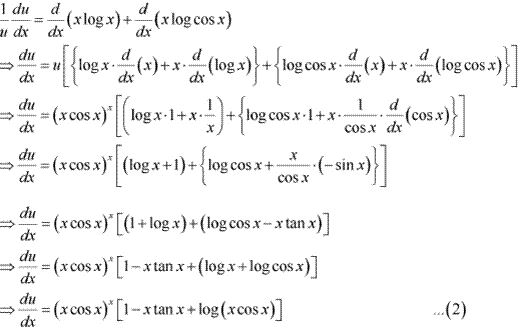v = (x sin x)1/x
⇒ log v = log (x sin x)1/x
⇒ log v = (1/x) log(x sin x)
⇒ log v = (1/x)[log x + log sin x]
⇒ log v = (1/x) log x + (1/x) log sin x
Differentiating both sides with respect to x, we obtain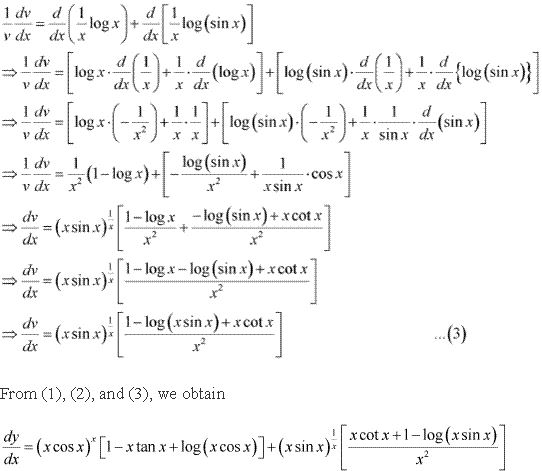12. Find dy/dx of function
xy + yx = 1
Solution
The given function is xy + yx = 1
Let xy = u and yx = v
Then, the function becomes u + v = 1
∴ du/dx + dv/dx = 0  ...(1)
u = xy
⇒ log u = log (xy )
⇒ log u = y log x
Differentiating both sides with respect to x, we obtain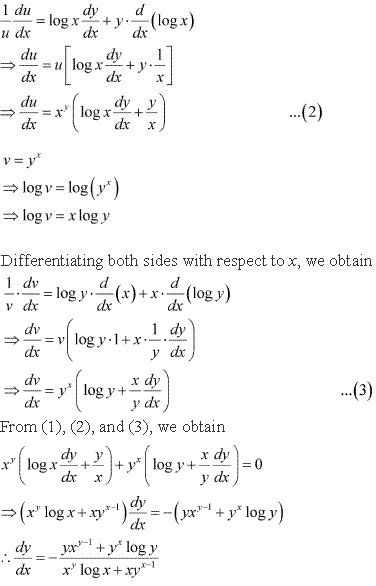13. Find dy/dx of function yx = xy
Solution
The given function is yx = xy
Taking logarithm on both the sides, we obtain
x log y = y log x
Differentiating both sides with respect to x, we obtain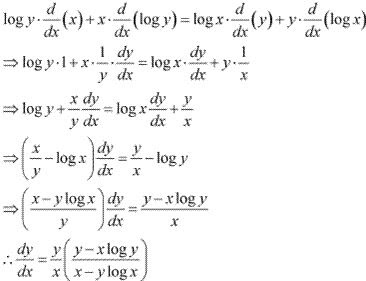14. Find dy/dx of function
(cos x)y + (cos y)x
Solution
The given function is (cos x)y + (cos y)x
Taking logarithm on both the sides, we obtain
y log cos x = x log cos y
differentiating both sides, we obtain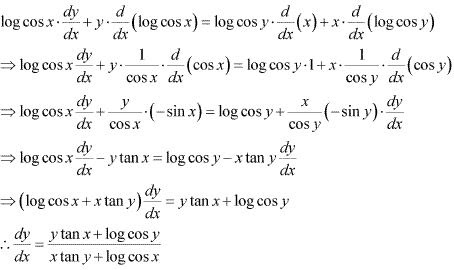15. Find dy/dx of function
xy = e(x - y)
Solution
The given function is xy = e(x - y)
Taking logarithm on both the sides, we obtain
log (xy) = log (ex - y )
⇒ log x + log y = (x - y) log e
⇒ log x + log y = (x - y) × 1
⇒ log x + log y = x - y
Differentiating both sides with respect to x, we obtain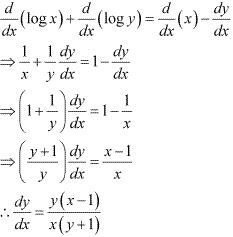16. Find the derivative of the function given by f(x) = (1 + x)(1 + x2 )(1 + x4 )(1 + x8 ) and hence find f '(1).
Solution
The given relationship is f (x) = (1 + x)(1 + x2 )(1 + x4 )(1 + x8 )
Taking logarithm on both the sides, we obtain
log f(x) = log (1 + x) + log(1 + x2 ) + log (1 + x4 ) + log(1 + x8 )
Differentiating both sides with respect to x, we obtain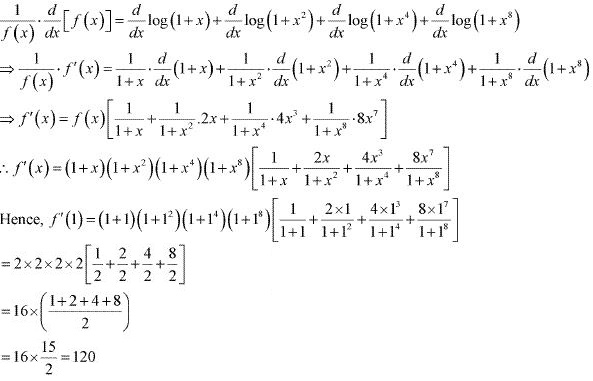17. Differentiate (x2 – 5x + 8) (x3 + 7x + 9) in three ways mentioned
(i) by using product rule
(ii) by expanding the product to obtain a single polynomial.
(iii) by logarithmic differentiation.
Do they all give the same answer?d below:
Solution
(i) Let x2 - 5x + 8 = u and x3 + 7x +9 = v
∴ y = uv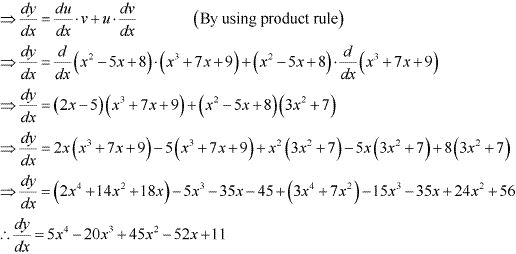(ii) y = (x2 - 5x + 8)(x3 + 7x + 9)
= x2 (x3 + 7x + 9) - 5x(x3 + 7x + 9) + 8(x2 + 7x + 9)
= x5 + 7x3 + 9x2 - 5x4 - 35x2 - 75x + 8x3 + 56x + 72
= x5 - 5x4 + 15x3 - 26x2 + 11x + 72
∴ dy/dx = d/dx (x5 - 5x4 + 15x3 - 26x2 + 11x + 72)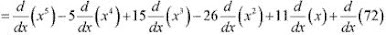= 5x4 - 5 × 4x3 + 15 × 3x2 - 26 × 2x + 11 × 1 + 0
= 5x4 - 20x3 + 45x2 - 52x + 11

(iii) y = (x2 - 5x + 8)(x3 + 7x + 9)
Taking logarithm on both the sides, we obtain
log y = log(x2 - 5x + 8) + log(x3 + 7x + 9)
Differentiating both sides with respect to x, we obtain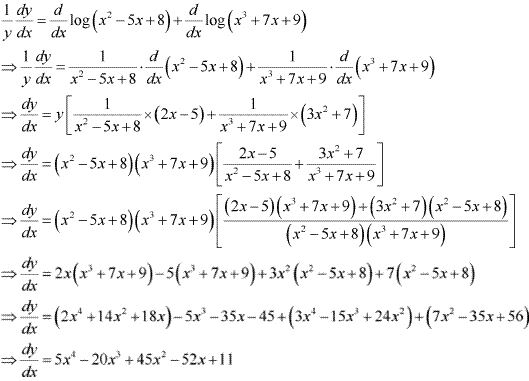18. If u, v and w are functions of x, then show that
d/dx(u. v. w) = du/dx (u.w) + u . (dv/dx) . w + u.v . dw/dx
in two ways - first by repeated application of product rule, second by logarithmic differentiation.
Solution
Let y = u.v.w = u.(v.w)
By applying product rule, we obtain